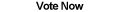Home Articles Components Code Snippets Links# Representing Graphs in C#

## Graphs

A graph is an extremely powerful data structure in computer science that gives rise to very powerful algorithms. Representing a graph in C# gives .NET programmers access to a wide variety of problem-solving algorithms and techniques. For example, graphs have applications in map processing, searching (like Google does), program compiling, and many many more fields.

We'll take a look at what graphs are in theory and how we can represent them in C#.

## What are Graphs

This article does not refer to plotting graphs. A graph data structure consists of two basic elements:

1. Vertex - A single node in the graph, often encapsulates some sort of information.
2. Edge - Connects one or two vertices. Can contain a value quantifying the weight of the edge.

A collection of vertices connected by edges is what is known as a graph in computer science. Graphs can the be categorized based on specific properties. Below is a general outline of the different types of graphs that exist:

• Undirected - edges connect vertices in no specific direction, two vertices are simply connected or not.
• Directed - edges connect vertices in a given direction, that is, vertex A might connect to vertex B, but vertex B does not connect to vertex A.
• Cyclic - contains at least one cycle (there exists a path starting at vertex A that eventually ends back at vertex A)
• Acyclic - a graph without cycles

These are the basic distinctions that can be made for graphs and many more exist. They are important because they tell us important information about the underlying data and the types of algorithms that can be performed on it. Some categories can also be combined, for example, a directed acyclic graph (DAG for short) is a special type of graph that is extremely important. An undirected acyclic graph is also known as a tree.

## Representing a Graph

There are several ways to represent a graph in a computer, here we will outline some basic representations.

The first way is with an adjacency list. In this representation, a graph consists of a collection of vertices. Each vertex keeps a list of its neighbors, i.e. vertices that have an edge that connects them to the given vertex. Note that in this case, an edge exist only conceptually, and is never explicitly defined.

```class Vertex<T>
{
private T data;
}```

As you can see this representation is extremely simple, which means it uses minimal memory. However as mentioned above, edges don't explicitly exist which can be a problem for some graph algorithms. To simplify our lives, we can make edges explicit at the cost of some additional memory.

```class Edge<T>
{
private Vertex<T> node1;
private Vertex<T> node2;
}

class Vertex<T>
{
private T data;
}```

Although each vertex is now redundantly referring itself in each edge, we gain an object that allows us to directly process edges in a graph. This is a concept that is very useful for graph algorithms, for example Kruskal's Algorithm goes through a graph's edges sorted by their weight. This form of representing graphs is sometimes referred to as an incident list.

A rather different approach is called an adjacency matrix. In this format, vertices exist by themselves and edges are stored in the form of a matrix. The matrix is n-by-n where n is the number of vertices in the graph. At each point (x,y) in the matrix, there is a value either 1 or 0 (true or false) marking if there exists an edge between vertex X and vertex Y.

```class Vertex<T>
{
private int index;
private T data;
}

class Graph<T>
{
List<Vertex<T>> vertices;
bool[][] edges = new bool;
}```

An immediate downside is the increased amount of memory required. Now edges are declared even when they don't exist. However this means we can find out whether an edge exists very quickly. We can also add and remove edges in constant time. This type of representation becomes very useful when dealing with graphs that have a lot of edges, and even more so if they have few vertices.

Each of these representations have their advantages and disadvantages. To leverage these accordingly, in C# we can create a Graph interface, and implement each of these representations extending that interface. Then graph algorithms can be written against the interface and the proper implementation can be used depending on what the algorithm does.

## Representing Vertices

There is one more wrinkle and that is, how we represent a vertex. As mentioned before, a vertex encapsulates data. But what data exactly? For an adjacency list we might need a list of neighbor vertices. For an adjacency matrix we need an integer index. How can we represent a vertex in C# if we don't know exactly what type of data it will hold?

Let's find out in part 2 - Representing Vertices in C# >

Back to C# Article List

Other C# Articles